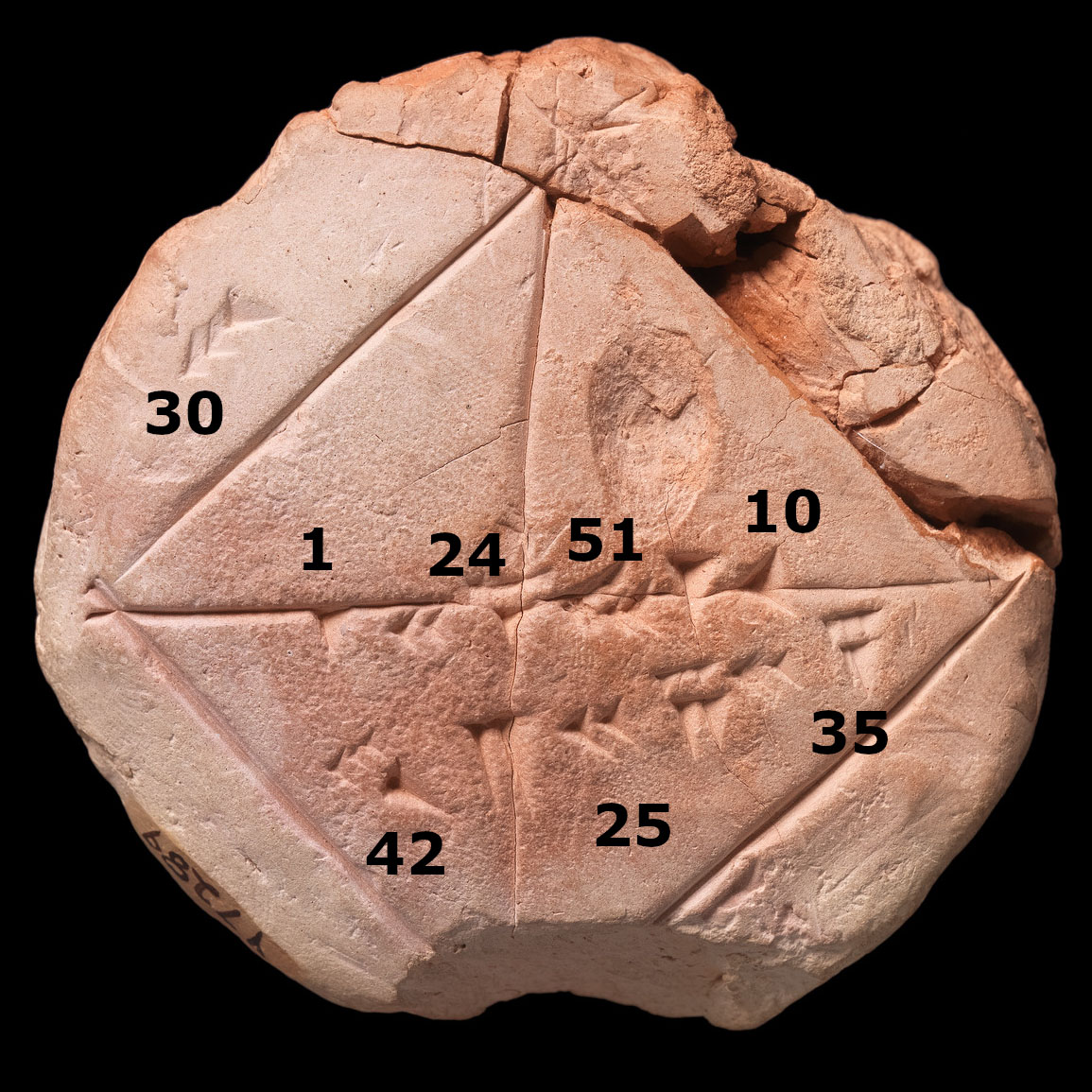Welcome to Project Lovelace! We're still in early development so there are still tons of bugs to find and improvements to make. If you have any suggestions, complaints, or comments please let us know on Discourse, Discord, or GitHub!

# Babylonian square roots

## You will learn about: calculating square roots, iterative algorithms, and Babylonian math.

The square root of a number S is denoted $\sqrt{S}$ with the property that $\sqrt{S} \times \sqrt{S} = S$. It's easy to calculate for square numbers, like $3 \times 3 = 9$ so $\sqrt{9} = 3$. For other numbers it's not as easy but the ancient Babylonians seemed to know how to calculate them pretty accurately (see the figure and caption).

We have no idea how they calculated square roots so accurately, but a method they might have used has been called the Babylonian method. The basic idea is to start with an initial guess $x_0$ for $\sqrt{S}$. Then we can keep improve on this guess: if our current guess, $x_n$, is an overestimate to $\sqrt{S}$ then $S/x_n$ will be an underestimate and vice versa, so averaging the two should produce a better guess: $$x_{n+1} = \frac{1}{2} \left( x_n + \frac{S}{x_n} \right)$$ We can use this formula to calculate better and better guesses. Given a number $S$, calculate and return its square root using the Babylonian method.A photograph of the Babylonian clay tablet YBC 7289. The numbers on the diagonal are actually a pretty accurate approximation of $\sqrt{2}$. They read in sexagesimal (base 60) 1, 24, 51, and 10 which cooresponds to $\sqrt{2} \approx 1 + 24/60 + 51/60^2 + 10/60^3 = 1.41421296...$ which is correct to six decimal digits! It also shows a square with sides of length 30 and diagonal of length $30 \sqrt{2} \approx 42 + 25/60 + 35/60^2$. (Source: Wikimedia Commons)

Input: A number $S \ge 0$.

Output: The square root of $S$ approximated using the babylonian method. Your answer should be accurate to 10 significant figures. That is, it should be correct to within 1 part in 10 billion, or the relative error should be lower than $10^{-10}$). You can calculate the relative error of your guess $x_n$ using $|x_n^2 - S| / S$ where $|\cdotp|$ is the absolute value (or abs) function.

Example

Input: 420 Output: 20.493901531919196
 Difficulty babylonian_sqrt(n)

You must be logged in to view your submissions.

### References

Babylon and the square root of 2, The Azimuth Project
Square Root Approximations in Old Babylonian Mathematics: YBC 7289 in Context , David Fowler & Eleanor Robson, Historia Mathematica, Volume 25, Issue 4, pp. 366-378 (1998).

Let us know what you think about this problem! Was it too hard? Difficult to understand? Also feel free to discuss the problem, ask questions, and post cool stuff on Discourse. You should be able see a discussion thread below. Would be nice if you don't post solutions in there but if you do then please organize and document your code well so others can learn from it.